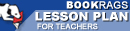Lesson Plans

# Daily Lessons for Teaching Prelude to Foundation

This set of Lesson Plans consists of approximately 90 pages of tests, essay questions, lessons, and other teaching materials.
 View a FREE sample## Lesson 1 (from "Mathematician" (pp. 1-24))

### Objective

PRELUDE TO FOUNDATION is the first book in the Foundation series.

The aim of this lesson is to examine the Foundation series.

### Lesson

1. Divide the class into groups and give each student the title of an Isaac Assimov Foundation novel to research. The students need to find out about the story, characters and some of the themes. What similarities do Assimov's Foundation novels share?

2. With the students brainstorm questions that will help them understand the book better. Ask the students to find the answers to the questions.

3. With the students brainstorm a typical scenario that could happen in a Foundation book. Divide the students into groups of three and assign each group a scenario. Their task is to write that scenario into a scene using the generic conventions from the Foundation books.

4. Homework. Read one of Asimov's short stories.

## Lesson 2 (from "Mathematician" (pp. 1-24))

### Objective

Hari Seldon is a mathematician...

(read more Daily Lessons)

 This section contains 4,484 words (approx. 15 pages at 300 words per page) View a FREE sampleMore summaries and resources for teaching or studying Prelude to Foundation.
CopyrightsPrelude to Foundation from BookRags. (c)2019 BookRags, Inc. All rights reserved.
Follow Us on Facebook